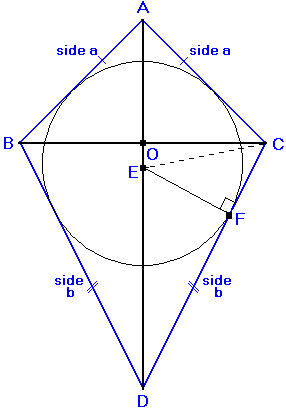## Right Kite Calculator

Select data you know.

Significant Figures >>>

All kites are tangential quadrilaterals, meaning that they are 4 sided figures into which a circle (called an incircle) can be inscribed such that each of the four sides will touch the circle at only one point. (Basically, this means that the circle is tangent to each of the four sides of the kite.)To inscribe a circle graphically (using compass and straight edge) within a kite:
• draw the axis of symmetry AD
• bisect one of the non-vertex angles (B or C) and extend this line so that it meets line AD at point E
• from point E, draw a perpendicular to any of the four sides.
• Inscribe the circle using point E as its center and line EF as its radius.

Describing this mathematically:
• Angle ACE = Angle ECD = ½ Angle ACD
• Angle ACO = 90º - ½ Angle BAC
• Angle OCE = Angle ACE - ACO
• OE = ½ Line BC x tan (OCE)
• AE = OA + OE